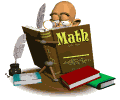•This year, your child will be working on the Houghton Mifflin California Math Curriculum.  Please note that this curriculum focuses on understanding concepts behind math.  You will note that there is always a written problem towards the bottem of their math page.  This is meant to help students verablize the concept in written form.   While this curriculum gives students review of computation, it is not something that students are asked to review, daily.  For this reason, we encourage students to continue fact practice at home.  Multiplication facts are a focus in third grade.  Please make sure to study these on a daily basis.  Students should have a great understanding of their multiplication facts by December, as they will be faced with division when they return from their holiday in January.  Below, you will find some links to practice pages. They are a great resource to use while practicing multiplication facts.    2's Multiplication Practice 3's Multiplication Practice 4's Multiplication Practice 5's Multiplication Practice 6's Multiplication Practice 7's Multiplication Practice 8's Multiplication Practice 9's Multiplication Practice 10's Multiplication Practice